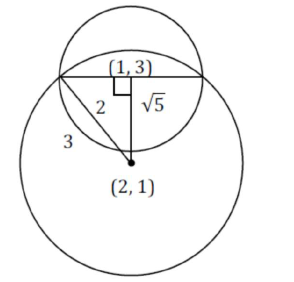# If one of the diameters o of the circleQuestion:

If one of the diameters o of the circle $x^{2}+y^{2}-2 x-6 y+6=0$ is a chord of another circle ${ }^{\prime} C^{\prime}$ whose center is at $(2,1)$, then its radius is

Solution:

distance between $(1,3)$ and $(2,1)$ is $\sqrt{5}$

$\therefore(\sqrt{5})^{2}+(2)^{2}=r^{2}$

$\Rightarrow r=3$